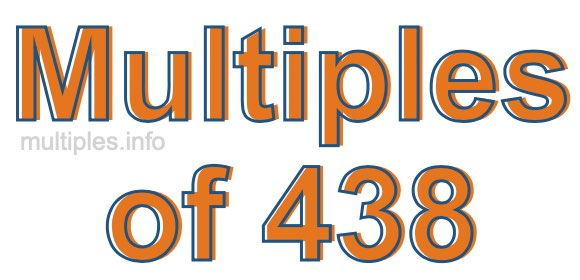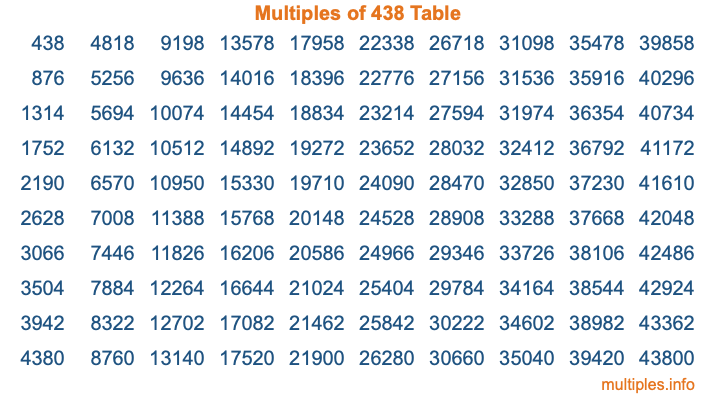Multiples of 438Welcome to the Multiples of 438 page. Here we will first teach you everything you will ever need to know about the multiples of 438, and then give you a study guide summary of everything we taught you to make sure you remember it all. Use this page to look up facts and learn information about the multiples of 438. This page will make you a multiples of four hundred thirty-eight expert!

Definition of Multiples of 438
Multiples of 438 are all the numbers that when divided by 438 equal an integer. Each of the multiples of 438 are called a multiple. A multiple of 438 is created by multiplying 438 by an integer.

Therefore, to create a list of multiples of 438, you start with 1 multiplied by 438, then 2 multiplied by 438, then 3 multiplied by 438, and so on for as long as you want. Thus, the list of the first five multiples of 438 is 438, 876, 1314, 1752, and 2190. To see a larger list of multiples of 438, see the printable image of Multiples of 438 further down on this page. We also have a category where you can choose any nth multiple of 438.

Multiples of 438 Checker
The Multiples of 438 Checker below checks to see if any number of your choice is a multiple of 438. In other words, it checks to see if there is any number (integer) that when multiplied by 438 will equal your number. To do that, we divide your number by 438. If the the quotient is an integer, then your number is a multiple of 438.

Is  a multiple of 438?

Least Common Multiple of 438 and ...
A Least Common Multiple (LCM) is the lowest multiple that two or more numbers have in common. This is also called the smallest common multiple or lowest common multiple and is useful to know when you are adding our subtracting fractions. Enter one or more numbers below (438 is already entered) to find the LCM.

Check out our LCM Calculator if you need more details about the Least Common Multiple or if you need the LCM for different numbers for adding and subtraction fractions.

nth Multiple of 438
As we stated above, 438 is the first multiple of 438, 876 is the second multiple of 438, 1314 is the third multiple of 438, and so on. Enter a number below to find the nth multiple of 438.

th multiple of 438

Multiples of 438 vs Factors of 438
438 is a multiple of 438 and a factor of 438, but that is where the similarities end. All postive multiples of 438 are 438 or greater than 438. All positive factors of 438 are 438 or less than 438.

Below is the beginning list of multiples of 438 and the factors of 438 so you can compare:

Multiples of 438: 438, 876, 1314, 1752, 2190, etc.

Factors of 438: 1, 2, 3, 6, 73, 146, 219, 438

As you can see, the multiples of 438 are all the numbers that you can divide by 438 to get a whole number. The factors of 438, on the other hand, are all the whole numbers that you can multiply by another whole number to get 438.

It's also interesting to note that if a number (x) is a factor of 438, then 438 will also be a multiple of that number (x).

Multiples of 438 vs Divisors of 438
The divisors of 438 are all the integers that 438 can be divided by evenly. Below is a list of the divisors of 438.

Divisors of 438: 1, 2, 3, 6, 73, 146, 219, 438

The interesting thing to note here is that if you take any multiple of 438 and divide it by a divisor of 438, you will see that the quotient is an integer.

Multiples of 438 Table
Below is an image of the first 100 multiples of 438 in a table. The table is in chronological order, column by column. The first column has the first ten multiples of 438, the second column has the next ten multiples of 438, and so on.The Multiples of 438 Table is also referred to as the 438 Times Table or Times Table of 438. You are welcome to print out our table for your studies.

Negative Multiples of 438
Although not often discussed or needed in math, it is worth mentioning that you can make a list of negative multiples of 438 by multiplying 438 by -1, then by -2, then by -3, and so on, to get the following list of negative multiples of 438:

-438, -876, -1314, -1752, -2190, etc.

Multiples of 438 Summary
Below is a summary of important Multiples of 438 facts that we have discussed on this page. To retain the knowledge on this page, we recommend that you read through the summary and explain to yourself or a study partner why they hold true.

There are an infinite number of multiples of 438.

A multiple of 438 divided by 438 will equal a whole number.

438 divided by a factor of 438 equals a divisor of 438.

The nth multiple of 438 is n times 438.

The largest factor of 438 is equal to the first positive multiple of 438.

438 is a multiple of every factor of 438.

438 is a multiple of 438.

A multiple of 438 divided by a divisor of 438 equals an integer.

438 divided by a divisor of 438 equals a factor of 438.

Any integer times 438 will equal a multiple of 438.

Multiples of a Number
Here you can get the multiples of another number, all with the same attention to detail as we did for multiples of 438 on this page.

Multiples of
Multiples of 439
Did you find our page about multiples of four hundred thirty-eight educational? Do you want more knowledge? Check out the multiples of the next number on our list!